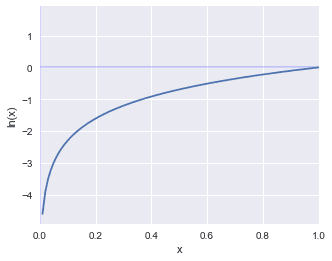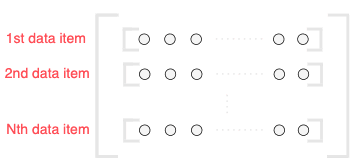search
Search
Publish
Guest 0reps
Thanks for the thanks!
close
Guides
Cancel
Post
account_circle
Profile
exit_to_app
Sign out
search
keyboard_voice
close
Searching Tips
Search for a recipe: "Creating a table in MySQL"
Search for an API documentation: "@append"
Search for code: "!dataframe"
Apply a tag filter: "#python"
Useful Shortcuts
/ to open search panel
Esc to close search panel
to navigate between search results
d to clear all current filters
Enter to expand content previewDoc SearchCode Search BetaSORRY NOTHING FOUND!
mic
Start speaking...Voice search is only supported in Safari and Chrome.
Shrink
Navigate to
A
A
share
thumb_up_alt
bookmark
arrow_backShareTwitterFacebook
Guides
thumb_up
0
thumb_down
0
chat_bubble_outline
0
auto_stories new
settings

# Neural Network | Cost functions

Machine Learning
chevron_right
Neural Networks
schedule Jul 1, 2022
Last updated
local_offer Machine LearningPython
Tags

A cost function (also sometimes referred to as loss or objective function) is used to quantify how well a model is performing. The lower the value of the cost function, the better the model is performing. In machine learning, the goal is to reduce the cost function as much as possible - this is what the training process is all about.

# Mean squared error

There are many types of cost functions that can be used, but the most well-known cost function is the mean squared error (abbreviated as $\mathrm{MSE}$):

$$\mathrm{MSE}=\frac{1}{2}\sum_k(y_k-t_k)^2$$

Where:

• $y_k$ is the element $k$ of the output (vector) of the neural network

• $t_k$ is the element $k$ of the true values

• $k$ is the number of dimensions of the data (the number of features or the size of the input vector)

## Implementation

Here, we define a function to compute the mean squared error given some predicted output $\mathrm{y}$ and an one-hot vector $\mathbf{t}$:

 import numpy as npdef mean_squared_error(y, t): return 0.5 * np.sum((y - t)**2)y = np.array([0.2, 0.4, 0.2, 0.1,0.1])t = np.array([0, 0, 1, 0, 0])mean_squared_error(y, t) 0.4300000000000001 

# Cross entropy error

The cross entropy error is defined as follows:

$$J=-\sum_kt_k\ln({y_k})$$

Where:

• $k$ the number of output neurons

• $t_k$ is the true label of the $k$-th neuron (either $0$ or $1$)

• $y_k$ is the output of the $k$-th neuron

## Example of manually computing cross entropy error

Suppose we have 3 output neurons. Suppose the output of the neurons is as follows:

$$\boldsymbol{y}= \begin{pmatrix} y_1\\y_2\\y_3 \end{pmatrix}= \begin{pmatrix} 0.2\\0.5\\0.3 \end{pmatrix}$$

The true labels is as follows:

$$\boldsymbol{t}= \begin{pmatrix} t_1\\t_2\\t_3 \end{pmatrix}= \begin{pmatrix} 0\\1\\0 \end{pmatrix}$$

Notice how the true label vector is an one-hot vector.

The cross entropy error can be computed like so:

\begin{align*} J&=-\sum_{k=1}^3t_k\ln({y_k})\\ &=-(t_1\ln({y_1})+t_2\ln({y_2})+t_3\ln({y_3}))\\ &=-((0)\ln({y_1})+(1)\ln({y_2})+(0)\ln({y_3}))\\ &=-\ln({y_2})\\ &=-\ln(0.5)\\ \end{align*}

The fact that $\boldsymbol{t}$ is a one-hot vector means that we are only interested in one slot in the vector $\boldsymbol{y}$. What this implies is that the summation doesn’t do very much here since every other elements will be zero anyway. This cost function only cares about how high the probability output of the class of interest is; the probability scores of the other classes are ignored.

## Intuition behind cross entropy error

Let’s further explore the behaviour of the cross entropy error. The following graph represents $y=\ln⁡(x)$:As we can see, if the $y_k=1$, then the cost will be $E=0$. An error term of $E=0$ is ideal since this means that the predicted label and the target label match. If the value of $y_k→0$, then $E=-\infty$ (i.e. just look at the graph). You may be wondering what would happen if $y_k\gt1$. Fortunately, we know for sure that $0\lt{y_k\lt1}$ since $y_k$ is the output of Softmax, and so there is nothing to worry about!

## Implementation

The implementation of cross entropy error is straight-forward:

 # y is a array of floats representing the output of Softmax# t is a one-hot array representing the true labelsdef cross_entropy_error(y, t): delta = 1e-7 return -np.sum(t * np.log(y + delta)) 

Here, we are using a very small number delta to account for the case when the $y=0$, which would make the log evaluate to negative infinity resulting in a math error.

Consider a good case when the predicted label - the label with highest computed probability - corresponds to the target label:

 y = np.array([0.1, 0.1, 0.1, 0.2, 0.4, 0, 0.1])t = np.array([ 0, 0, 0, 0, 1, 0, 0])print(cross_entropy_error(y, t)) 0.9162904818741863 

Consider a bad case when the predicted label does not correspond to the target label:

 y = np.array([0.1, 0.1, 0.1, 0.5, 0.1, 0, 0.1])t = np.array([ 0, 0, 0, 0, 1, 0, 0])print(cross_entropy_error(y, t)) 2.302584092994546 

Observe how the entropy error is larger for the bad case, just as you would expect.

# Mini-batch training

In order to compute the cost function $E$, we need to take the average cost function of $M$ data items , that is, the entire training set. Therefore, if we have $1000$ training data items, then we would need to compute the cost function $1000$ times, and then take the average.

This process works well if the size of the training set is small, yet becomes drastically slow for large data sets. In order to overcome this problem, we introduce the concept of mini-batch training, The concept is simple; instead of taking the entire $M$ data items, we just take some small portion $N$ randomly from $M$ data items.

$$E=-\frac{1}{N}\sum_n\sum_k{t_{nk}}\ln(y_{nk})$$

Where:

• $N$ is the chosen batch size

• $t_{nk}$ is the element $k$ of one-hot vector (true label) for the selected batch item $n$

• $y_{nk}$ is the element $k$ of the output of Softmax for the selected batch item $n$

The idea is to sample from the population of data items. The data items that are randomly sampled are called mini-batch.

## Implementation

Suppose we have the following training set:

 X_train = np.random.random([5, 3])X_train array([[0.1086232 , 0.61294663, 0.84318942], [0.46938155, 0.80033484, 0.47092242], [0.17128571, 0.4384816 , 0.96025601], [0.29355096, 0.45201871, 0.71259535], [0.62011513, 0.50227353, 0.67432293]]) 

Define a helper function to extract random rows from X_train:

 def get_mini_batch(X_train): BATCH_SIZE = 3 train_size = X_train.shape # Sample without replacement batch_index = np.random.choice(train_size, BATCH_SIZE) return X_train[batch_index] 

We then call our function like so:

 get_mini_batch(X_train) array([[0.62011513, 0.50227353, 0.67432293], [0.17128571, 0.4384816 , 0.96025601], [0.29355096, 0.45201871, 0.71259535]]) 

Schematically, our mini-batch array looks like the following:## Implementing cross entropy error for mini-batch

For mini-batch training, the code to compute the cross entropy error is as follows:

 # y is a array of floats representing the output of Softmax# t is a one-hot array representing the true labelsdef cross_entropy_error(y, t): if y.ndim == 1: # Convert vector into matrix t = np.reshape(t, (1, t.size)) y = np.reshape(y, (1, y.size)) batch_size = y.shape delta = 1e-7 # Here, the sum computes the sum of the 2D array (returns a scalar) return -np.sum(t * np.log(y + delta)) / batch_size